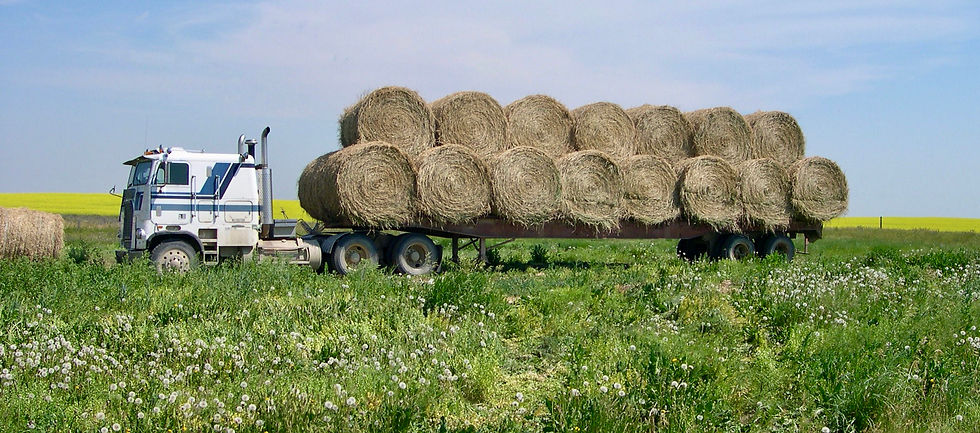top of page

# Calculating the Value of Hay Based on Moisture Content

StettlerLocal.com Aug 10, 2022 @ 7:00pmIt is common practice for hay to be traded by the bale. Unfortunately, bales that appear identical can vary in weight by as much as 200 pounds. Weighing a couple of loads of hay will provide an average weight. Standard moisture content in hay bales is considered to be 16%. Therefore, knowing the bale weight and using a standard moisture content is enough information to come to an agreement on bale price.

What happens if the moisture in the bale is higher or lower than the standard 16%? Using straight math, if the moisture content is 3% higher, the price is adjusted down by 3%. Using \$70 per ton as the agreed to price, this calculation would result in an adjusted price of \$67.90 per ton. Unfortunately, this is not correct.

To properly calculate a price adjustment, it is necessary to calculate the weight of dry hay per ton for both moisture levels. The second step is to convert the price of the hay to a dry basis. Thirdly, multiply the pounds of dry matter per ton of the wetter hay by the value of the drier hay on a moisture-free basis.

In this example, a ton (2,000 pounds) of hay contains 16% moisture.

Multiply 2000 pounds x 84% dry matter = 1,680 pounds of dry matter per ton.

Divide the value per ton by the number of pounds of dry matter to get a hay value on a dry matter basis. \$70 per ton divided by 1680 pounds = 4.16 cents a pound.

This is the base value for the hay on a dry matter basis.

If moisture is greater, 19 %, for example, calculate the amount of dry matter per ton. Then, multiply this value by the base value of 4.16 cents a pound to obtain the price per ton.

2000 pounds x 81% dry matter = 1,620 pounds of dry matter per ton.

1620 pounds x 4.16 cents a pound = \$67.39 per ton.

The difference in calculation methods is \$0.51 per ton.

The same process can be used for drier hay and between hay and silage. First, calculate pounds of dry matter and then multiply by the value per pound. This methodology is mathematically correct and can be used for grain as well.Barry Yaremcio

Ruminant Nutritionist

See All
bottom of page